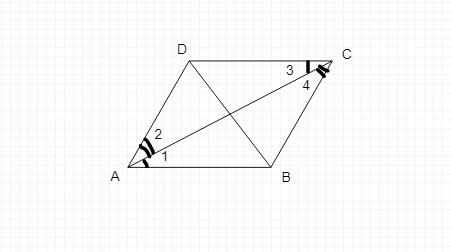# Prove that, in a parallelogram1)opposite sides are equal 2) opposite angles are equal 3) Each diagonal will divide the parallelogram into two congruent triangles

Given :

We have to prove that , in a parallelogram,

1)opposite sides are equal

2) opposite angles are equal

3) Each diagonal will divide the parallelogram into two congruent triangles

Solution :

Take a parallelogram ABCD and join

Two of its non-adjacent vertices, say A and C.In the parallelogram ABCD,

BC || AD and AB || DC.

AC is the transversal between the parallel lines BC and AD, and it is also a transversal between the parallel lines AB and DC.

Let the angles formed by these parallel lines and transversal be angles 1, 2, 3 and 4.

Consider ∆ ABC and ∆ CDA,

1 = 3         (since alternate angles are equal)

2 = 4         (since alternate angles are equal)

AC = CA          (common side)

So, by the ASA rule ∆ ABC CDA.

Therefore, diagonal AC divides

parallelogram ABCD into two congruent triangles ABC and CDA.

In congruent triangles ABC and CDA,

BC = DA     (corresponding sides of congruent triangles)

Similarly, BA = DC.

BC and DA; BA and DC are opposite sides in the parallelogram ABCD.

Therefore, in a parallelogram, opposite sides are equal.

Hence proved.

Updated on: 10-Oct-2022

38 Views